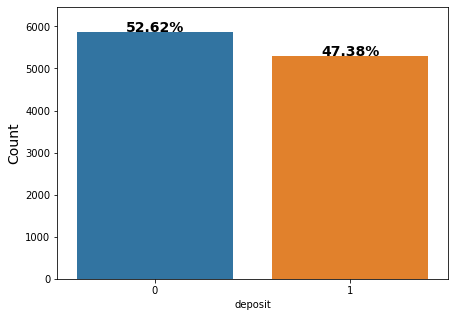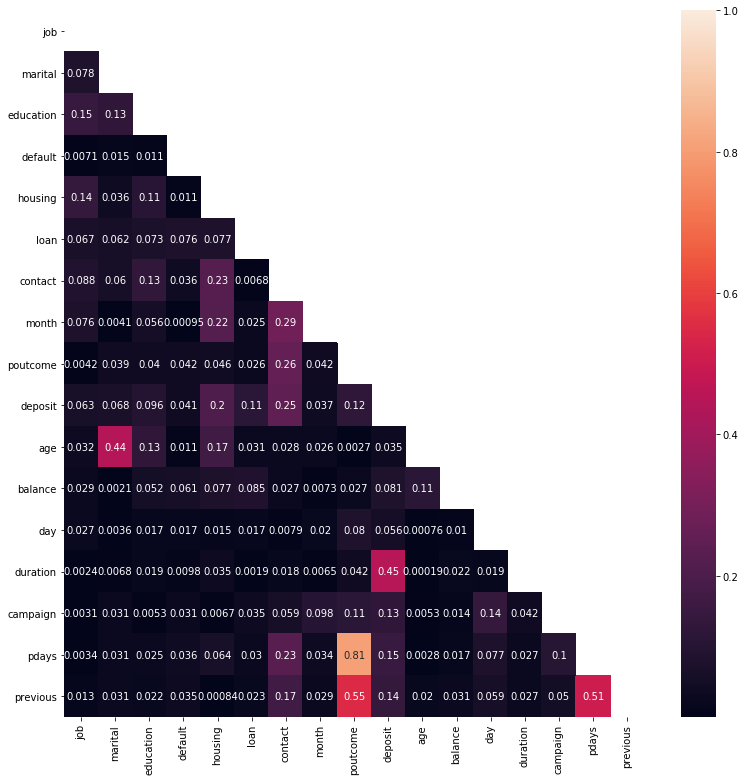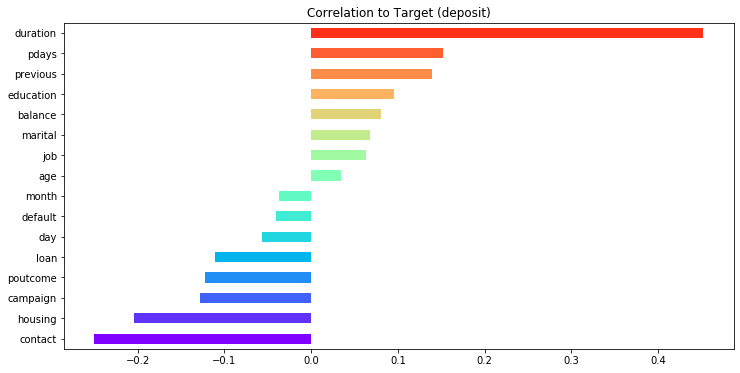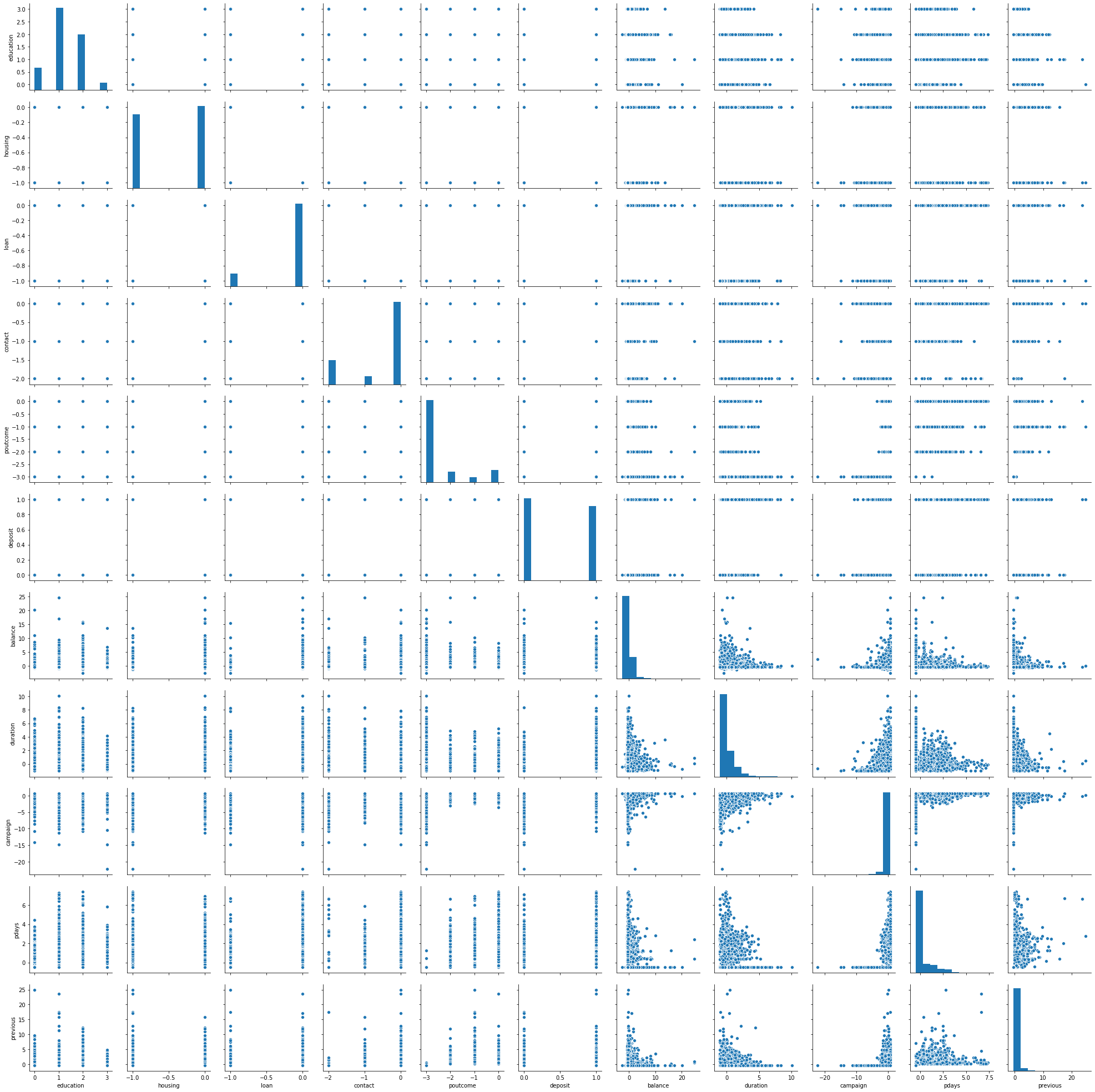# The PyMC3 workflow¶

PyMC3 aims for intuitive and readable, yet powerful syntax that reflects how statisticians describe models. The modeling process generally follows these five steps:

1. Encode a probability model by defining the following:
1. The prior distributions that quantify knowledge and uncertainty about latent variables
2. The likelihood function that conditions the parameters on observed data
1. Analyze the posterior using one of the options:
1. Obtain a point estimate using MAP inference
2. Sample from the posterior using MCMC methods
1. Approximate the posterior using variational Bayes.
2. Check the model using various diagnostic tools.
3. Generate predictions.

The resulting model can be used for inference to gain detailed insights into parameter values as well as to predict outcomes for new data points.

# Logistic regression with PyMC3¶

Logistic regression estimates a linear relationship between a set of features and a binary outcome, mediated by a sigmoid function to ensure the model produces probabilities. The frequentist approach resulted in point estimates for the parameters that measure the influence of each feature on the probability that a data point belongs to the positive class, with confidence intervals based on assumptions about the parameter distribution.

In contrast, Bayesian logistic regression estimates the posterior distribution over the parameters itself. The posterior allows for more robust estimates of what is called a Bayesian credible interval for each parameter with the benefit of more transparency about the model’s uncertainty.

## Imports¶

In :
import warnings
warnings.filterwarnings('ignore')

In :
%matplotlib inline
from pathlib import Path
import pickle
from collections import OrderedDict
import pandas as pd
import numpy as np
from scipy import stats

from sklearn import preprocessing
from sklearn.model_selection import train_test_split
from sklearn.metrics import (roc_curve, roc_auc_score, confusion_matrix, accuracy_score, f1_score,
precision_recall_curve)
from mlxtend.plotting import plot_confusion_matrix

import theano
import pymc3 as pm
from pymc3.variational.callbacks import CheckParametersConvergence
import statsmodels.formula.api as smf

import arviz as az
import matplotlib.pyplot as plt
import matplotlib.cm as cm

import seaborn as sns
from IPython.display import HTML

In :
data_path = Path('data')
fig_path = Path('figures')
model_path = Path('models')
for p in [data_path, fig_path, model_path]:
if not p.exists():
p.mkdir()


## The Data¶

The goal of this dataset is to create a binary classification model that predicts whether or not over $11,000$ customers will subscribe to a term deposit after a marketing campaign the bank has performed, based on $17$ predictor variables. The target variable is given as deposit and takes on a value of $1$ if the customer has subscribed and $0$ otherwise.

This is a slightly imbalanced class problem because there are more customers that did not subscribe the term deposit than did.

In :
data = pd.read_csv('bank.csv')
data.info()

<class 'pandas.core.frame.DataFrame'>
RangeIndex: 11162 entries, 0 to 11161
Data columns (total 17 columns):
age          11162 non-null int64
job          11162 non-null object
marital      11162 non-null object
education    11162 non-null object
default      11162 non-null object
balance      11162 non-null int64
housing      11162 non-null object
loan         11162 non-null object
contact      11162 non-null object
day          11162 non-null int64
month        11162 non-null object
duration     11162 non-null int64
campaign     11162 non-null int64
pdays        11162 non-null int64
previous     11162 non-null int64
poutcome     11162 non-null object
deposit      11162 non-null object
dtypes: int64(7), object(10)
memory usage: 1.4+ MB

In :
data.head()

Out:
age job marital education default balance housing loan contact day month duration campaign pdays previous poutcome deposit
0 59 admin. married secondary no 2343 yes no unknown 5 may 1042 1 -1 0 unknown yes
1 56 admin. married secondary no 45 no no unknown 5 may 1467 1 -1 0 unknown yes
2 41 technician married secondary no 1270 yes no unknown 5 may 1389 1 -1 0 unknown yes
3 55 services married secondary no 2476 yes no unknown 5 may 579 1 -1 0 unknown yes
4 54 admin. married tertiary no 184 no no unknown 5 may 673 2 -1 0 unknown yes

### Data Preprocessing¶

In :
categorical_features = data.select_dtypes(include=[np.object])

numeric_features = data.select_dtypes(include=[np.number])

cols = numeric_features.columns
numeric_features.loc[:, cols] = preprocessing.scale(numeric_features.loc[:, cols])

le = preprocessing.LabelEncoder()

categorical_features = data[categorical_features.columns].apply(lambda x: le.fit_transform(x))

data = pd.concat([categorical_features, numeric_features], axis=1)
data.info()

<class 'pandas.core.frame.DataFrame'>
RangeIndex: 11162 entries, 0 to 11161
Data columns (total 17 columns):
job          11162 non-null int64
marital      11162 non-null int64
education    11162 non-null int64
default      11162 non-null int64
housing      11162 non-null int64
loan         11162 non-null int64
contact      11162 non-null int64
month        11162 non-null int64
poutcome     11162 non-null int64
deposit      11162 non-null int64
age          11162 non-null float64
balance      11162 non-null float64
day          11162 non-null float64
duration     11162 non-null float64
campaign     11162 non-null float64
pdays        11162 non-null float64
previous     11162 non-null float64
dtypes: float64(7), int64(10)
memory usage: 1.4 MB

In :
data.head()

Out:
job marital education default housing loan contact month poutcome deposit age balance day duration campaign pdays previous
0 0 1 1 0 1 0 2 8 3 1 1.491505 0.252525 -1.265746 1.930226 -0.554168 -0.481184 -0.36326
1 0 1 1 0 0 0 2 8 3 1 1.239676 -0.459974 -1.265746 3.154612 -0.554168 -0.481184 -0.36326
2 9 1 1 0 1 0 2 8 3 1 -0.019470 -0.080160 -1.265746 2.929901 -0.554168 -0.481184 -0.36326
3 7 1 1 0 1 0 2 8 3 1 1.155733 0.293762 -1.265746 0.596366 -0.554168 -0.481184 -0.36326
4 0 1 2 0 0 0 2 8 3 1 1.071790 -0.416876 -1.265746 0.867171 -0.186785 -0.481184 -0.36326

### Quick EDA¶

In :
total = len(data)
plt.figure(figsize=(7,5))
g = sns.countplot(x='deposit', data=data)
g.set_ylabel('Count', fontsize=14)
for p in g.patches:
height = p.get_height()
g.text(p.get_x()+p.get_width()/2.,
height + 1.5,
'{:1.2f}%'.format(height/total*100),
ha="center", fontsize=14, fontweight='bold')
plt.margins(y=0.1)
plt.show()In :
plt.figure(figsize=(13, 13))
corr = data.corr()
b, t = plt.ylim()
b += 0.5
t -= 0.5
plt.ylim(b, t)
plt.show()In :
n_fts = len(data.columns)
colors = cm.rainbow(np.linspace(0, 1, n_fts))

data.drop('deposit',axis=1).corrwith(data.deposit).sort_values(ascending=True).plot(kind='barh',
color=colors, figsize=(12, 6))
plt.title('Correlation to Target (deposit)')
plt.show()

print('\n',data.drop('deposit',axis=1).corrwith(data.deposit).sort_values(ascending=False))duration     0.451919
pdays        0.151593
previous     0.139867
education    0.095948
balance      0.081129
marital      0.067610
job          0.063395
age          0.034901
month       -0.037121
default     -0.040680
day         -0.056326
loan        -0.110580
poutcome    -0.122369
campaign    -0.128081
housing     -0.203888
contact     -0.249847
dtype: float64

In :
data['housing'] = -data['housing']
data['contact'] = -data['contact']
data['campaign'] = -data['campaign']
data['poutcome'] = -data['poutcome']
data['loan'] = -data['loan']

corr = data.corr()

cor_target = corr["deposit"]

relevant_features = cor_target[cor_target > 0.08]
relevant_features.sort_values(ascending=False)

Out:
deposit      1.000000
duration     0.451919
contact      0.249847
housing      0.203888
pdays        0.151593
previous     0.139867
campaign     0.128081
poutcome     0.122369
loan         0.110580
education    0.095948
balance      0.081129
Name: deposit, dtype: float64
In :
sns.pairplot(data[relevant_features.index]);### Models¶

In :
simple_model = 'deposit ~ duration + pdays + previous'
full_model = 'deposit ~ duration + pdays + previous + education + balance +  loan + poutcome + campaign +  housing + contact'


## MAP Inference¶

A probabilistic program consists of observed and unobserved random variables (RVs). We define the observed RVs via likelihood distributions and unobserved RVs via prior distributions. PyMC3 includes numerous probability distributions for this purpose.

The PyMC3 library makes it very straightforward to perform approximate Bayesian inference for logistic regression. Logistic regression models the probability that individual $i$ subscribes to a deposit based on $k$ features.

Logistic Regression:

$$p(y_i = 1 \mid \beta) = \sigma \beta_0 + \beta_1 x_{i1} + \dots + \beta_k x_{ik})$$

Where $\sigma$ is the logistic function: $$\sigma(t) = \frac{1}{1 + e^{-1}}$$

### Manual Model Specification¶

We will use the context manager with to define a manual_logistic_model that we can refer to later as a probabilistic model:

1. The random variables for the unobserved parameters for intercept and $3$ features are expressed using uninformative priors that assume normal distributions with mean $0$ and standard deviation of $100$.
1. The likelihood combines the parameters with the data according to the specification of the logistic regression.
1. The outcome is modeled as a Bernoulli RV with success probability given by the likelihood.
In :
with pm.Model() as manual_logistic_model:
# random variables for coefficients with
# uninformative priors for each parameter

intercept = pm.Normal('intercept', 0, sd=100)
beta_1 = pm.Normal('beta_1', 0, sd=100)
beta_2 = pm.Normal('beta_2', 0, sd=100)
beta_3 = pm.Normal('beta_3', 0, sd=100)

# Transform random variables into vector of probabilities p(y_i=1)
# according to logistic regression model specification.
likelihood = pm.invlogit(intercept + beta_1 * data.duration + beta_2 * data.pdays + beta_3 * data.previous)

# Bernoulli random vector with probability of success
# given by sigmoid function and actual data as observed
pm.Bernoulli(name='logit', p=likelihood, observed=data.deposit)

In :
manual_logistic_model.model

Out:
$$\begin{array}{rcl} \text{intercept} &\sim & \text{Normal}(\mathit{mu}=0.0,~\mathit{sigma}=100.0)\\\text{beta_1} &\sim & \text{Normal}(\mathit{mu}=0.0,~\mathit{sigma}=100.0)\\\text{beta_2} &\sim & \text{Normal}(\mathit{mu}=0.0,~\mathit{sigma}=100.0)\\\text{beta_3} &\sim & \text{Normal}(\mathit{mu}=0.0,~\mathit{sigma}=100.0)\\\text{logit} &\sim & \text{Bernoulli}(\mathit{p}=f(f(f(),~f(f(),~f(f(f(f(f(f(\text{intercept}),~f(f(\text{beta_1}),~array)),~f(f(\text{beta_2}),~array)),~f(f(\text{beta_3}),~array)))))),~f())) \end{array}$$

The command pm.model_to_graphviz(manual_logistic_model) produces the plate notation displayed below.

It shows the unobserved parameters as light and the observed elements as dark circles. The rectangle indicates the number of repetitions of the observed model element implied by the data included in the model definition.

In :
pm.model_to_graphviz(manual_logistic_model)

Out:

### MAP Estimate¶

Maximum a posteriori probability (MAP) estimation leverages that the evidence is a constant factor that scales the posterior to meet the requirements for a probability distribution. Since the evidence does not depend on $θ$, the posterior distribution is proportional to the product of the likelihood and the prior. Hence, MAP estimation chooses the value of $θ$ that maximizes the posterior given the observed data and the prior belief, that is, the mode of the posterior.

$$\theta_{MAP} = \operatorname*{arg\,max}_\theta P(X \mid \theta) P(\theta)$$

We obtain point MAP estimates for the three parameters using the just defined model’s .find_MAP() method:

In :
with manual_logistic_model:
# compute maximum a-posteriori estimate
# for logistic regression weights
manual_map_estimate = pm.find_MAP()

logp = -7,759, ||grad|| = 2,784: 100%|██████████| 11/11 [00:00<00:00, 450.08it/s]

In :
def print_map(result):
return pd.Series({k: np.asscalar(v) for k, v in result.items()})

In :
print_map(manual_map_estimate)

Out:
intercept    0.051369
beta_1       1.561395
beta_2       0.260651
beta_3       0.306804
dtype: float64

### GLM Model¶

PyMC3 includes numerous common models so that we can usually leave the manual specification for custom applications.

The following code defines the same logistic regression as a member of the Generalized Linear Models (GLM) family using the formula format inspired by the statistical language R and ported to python by the patsy library:

In :
with pm.Model() as logistic_model:
pm.glm.GLM.from_formula(simple_model,
data,
family=pm.glm.families.Binomial())

In :
pm.model_to_graphviz(logistic_model)

Out:

PyMC3 solves the optimization problem of finding the posterior point with the highest density using the quasi-Newton Broyden-Fletcher-Goldfarb-Shanno (BFGS) algorithm but offers several alternatives provided by the scipy library. The result is virtually identically to the corresponding statsmodels estimate:

In :
model = smf.logit(formula=simple_model, data=data[['deposit', 'duration', 'pdays', 'previous']])
result = model.fit()
print(result.summary())

Optimization terminated successfully.
Current function value: 0.538611
Iterations 6
Logit Regression Results
==============================================================================
Dep. Variable:                deposit   No. Observations:                11162
Model:                          Logit   Df Residuals:                    11158
Method:                           MLE   Df Model:                            3
Date:                Thu, 07 May 2020   Pseudo R-squ.:                  0.2214
Time:                        17:55:19   Log-Likelihood:                -6012.0
converged:                       True   LL-Null:                       -7721.6
Covariance Type:            nonrobust   LLR p-value:                     0.000
==============================================================================
coef    std err          z      P>|z|      [0.025      0.975]
------------------------------------------------------------------------------
Intercept      0.0514      0.023      2.192      0.028       0.005       0.097
duration       1.5614      0.037     42.443      0.000       1.489       1.633
pdays          0.2607      0.027      9.816      0.000       0.209       0.313
previous       0.3068      0.032      9.585      0.000       0.244       0.370
==============================================================================

In :
print_map(manual_map_estimate)

Out:
intercept    0.051369
beta_1       1.561395
beta_2       0.260651
beta_3       0.306804
dtype: float64
In :
result.params

Out:
Intercept    0.051370
duration     1.561394
pdays        0.260652
previous     0.306804
dtype: float64

## Markov Chain Monte Carlo¶

Markov chains are stochastic models that describe sequences of possible events. Each event comes from a set of outcomes, and each outcome determines which outcome occurs next, according to a fixed set of probabilities. An important feature of Markov chains is that they are memoryless: everything that you would possibly need to predict the next event is available in the current state, and no new information comes from knowing historical events.

Monte Carlo methods rely on repeated random sampling to approximate results that may be deterministic, but that does not permit an analytic, exact solution.

Many algorithms apply the Monte Carlo method to a Markov Chain, and generally proceed as follows:

1. Start at the current position.
2. Draw a new position from a proposal distribution.
3. Evaluate the probability of the new position in light of data and prior distributions:
1. If sufficiently likely, move to the new position
2. Otherwise, remain at the current position
4. Repeat from step 1.
5. After a given number of iterations, return all accepted positions.

### Define the Model¶

We will use a slightly more complicated model to illustrate Markov chain Monte Carlo inference:

• full_model = 'deposit ~ duration + pdays + previous + education + balance + loan + poutcome + campaign + housing + contact'
In :
with pm.Model() as logistic_model:
pm.glm.GLM.from_formula(formula=full_model,
data=data,
family=pm.glm.families.Binomial())

In :
logistic_model.basic_RVs

Out:
[Intercept,
duration,
pdays,
previous,
education,
balance,
loan,
poutcome,
campaign,
housing,
contact,
y]

### Hamiltonian Monte Carlo – going NUTS¶

By default, PyMC3 automatically selects the most efficient sampler and initializes the sampling process for efficient convergence. For a continuous model, PyMC3 chooses the NUTS sampler. It also runs variational inference via ADVI to find good starting parameters for the sampler. One among several alternatives is to use the MAP estimate.

Hamiltonian Monte Carlo (HMC) is a hybrid method that leverages the first-order derivative information of the gradient of the likelihood to propose new states for exploration and overcome some of the challenges of MCMC. In addition, it incorporates momentum to efficiently jump around the posterior. As a result, it converges faster to a high-dimensional target distribution than simpler random-walk Metropolis or Gibbs sampling.

To see what the convergence looks like, we first draw $1,000$ samples after tuning the sampler for $1,000$ iterations that will be discarded. The sampling process can be parallelized for multiple chains using the cores argument (except when using GPU).

In :
with logistic_model:
trace = pm.sample(tune=1000,
draws=1000,
chains=4,
cores=3)

Auto-assigning NUTS sampler...

pm.plot_trace(trace);